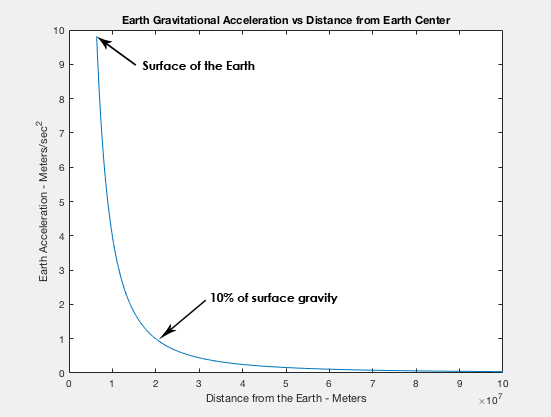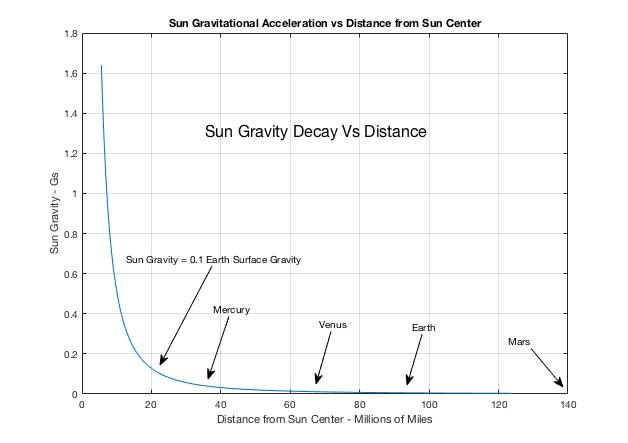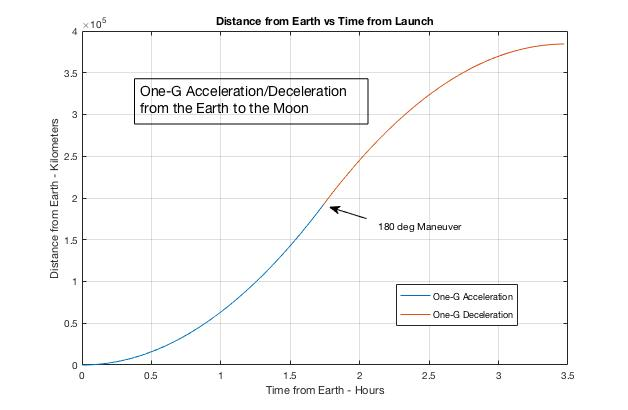# Astrodynamics Primer

Human Spaceflight in 2070

To understand space travel about the Solar System in 2070 as presented in Robot Dawn, it is necessary to understand the equivalence of gravity and acceleration. While standing on the surface of the Earth, we experience gravity as a force trying to pull us downward. The Earth provides a force acting upward on our feet that resists gravity. Thus, we are put in a state of equilibrium. If we are floating in outer space far from any large  body, we experience weightlessness and float free. If we are standing in a rocket ship that is accelerating, we will be forced against the floor and will experience it as gravity. If the acceleration is equal to one-g, 9.8 meters/sec^2 (32.2 ft/sec^2), we will experience standing on the floor of the rocket ship with it pushing against us the same as standing on Earth. We can’t tell the difference. This means that if we have a rocket ship that can provide one-g continuously, we will have the same experience as we would on the surface of the Earth.

The way we currently (2018) get from place to place within the Solar System is to provide an initial acceleration that gives sufficient velocity for us to drift along in an orbit until we encounter our destination where we provide a decelerating force to slow us so we can land.  That means that during the takeoff thrust/acceleration phase and the landing thrust/deceleration phase, we experience accelerations that far exceed that of one-g. In between the takeoff and landing phases, we experience zero-g weightlessness for an extended period of time.

Prolonged weightlessness of astronauts in space is perhaps the most serious problem that effects them both physically and emotionally even beyond the initial “seasickness” many experience. Weightlessness causes a loss of calcium that weakens the bones, and muscles also lose mass. If our space ship always traveled with an acceleration of one-g, these problems would not occur. One-g acceleration is perfect for making all passengers comfortable during flight, but one-g acceleration also has many benefits in getting around in the Solar System. It rapidly creates high velocities, and enables astronauts to get where they are going quickly. Traveling to the Moon would be like going from San Francisco to Los Angeles by automobile. Going to Mars would be like traveling from San Francisco to New York City by train.

This elementary dissertation explains how space travel has been made more comfortable for human passengers since the invention of Cold Fusion (CF) power and Electromagnetic Drive (EmDrive) propulsion in the decades prior to 2070. This was accomplished by providing a one-g environment throughout the duration of spaceflight. The most startling result is that we no longer have to go into orbit about a body or use Hohmann transfer orbits to get from one heavenly body to another in the Solar System. We can just brute-force it as long as we are outside the gravity-well of large Solar System objects such as the Sun, Earth, Moon and other planets and their moons. The gravitational force falls off as the square of the distance from the body, which means that the gravity-well is much smaller than might be expected. This makes it much easier to negotiate the Solar System than even a knowledgeable person might think . The reason for this is that a vehicle acceleration of one-g is a good healthy acceleration, and the velocities that can be attained in a relative short period make the Solar System seem like it is simply part of our own backyard. One-g acceleration for one hour results in a velocity of 130,000 km/hour (80,000 miles/hour). One-g acceleration for one year can get you close to the speed of light, 300,000 km/sec (186,000 miles/sec), and far outside the Solar System, even beyond the Oort Cloud.

Even though gravity falls off with the square of the distance from the body, it never is zero. Because of this, we must define the point where the gravity-well no longer is of major importance. I have defined that point as being one-tenth of Earth’s gravitational acceleration at the surface. The reason for this definition is that a human being can tolerate an apparent increase of 10% in their own body weight without experiencing much discomfort. Go above 10%, and normal activities become more of a struggle. This point is the edge of gravity-well primary human influence. I have termed it the Ten-Percent Zone or TPZone. Here are some TPZones for Solar System objects:

Solar TPZone = 0.08 au from the center of the Sun. Just a little less than 10% from the Sun’s center to the Earth.
Lunar TPZone = 1.28 % of the Moon’s radius. Just 306 miles off the surface of the Moon.
Earth TPZone = 12,600 miles (20,300 km) from the center of the Earth.

(The TPZone should not be confused with the Hill sphere, which approximates the gravitational sphere of influence of a smaller body in the face of perturbations from a more massive body.)

Of course, once passengers have reached their destination, they will be subjected to local gravitation, which usually is much different from one-g. If the destination is to orbit about another heavenly body, the passenger will experience zero-g, or as it is now termed, microgravity. However, “artificial” gravity can be provided through centripetal force by spinning all or a portion of a structure, as for instance the two space colonies at EM-L4 and EM-L5. I cover that in another post. Click here.

This astrodynamics primer is based of the following assumptions:

1. Within the Solar System, humans should always travel under the influence of constant one-g acceleration, which simulates conditions on the surface of the Earth under which humans evolved.
2. Space vehicles in 2070 have a Cold Fusion power source that provides as much power as needed instantaneously.
3. Space vehicles have Electromagnetic Drives (EmDrives) that provide constant one-g acceleration without ejecting reaction propellant for the duration of flight that would pollute the Solar System due to the heavy traffic about it in 2070.

ATMOSPHERIC FLIGHT ASCENT TO SPACE

When an EmDrive spacecraft leaves the surface of the Earth heading into outer space, it goes straight up. Since the density of the atmosphere is reduced to 1.0 percent at 100,000 feet (~20 miles), the spacecraft can go from the surface of the Earth to the top of the atmosphere at a constant 60 mph in 20 minutes. Therefore, the shape of the vehicle is of minimal importance for aerodynamic drag, and the buildup of dynamic pressure is also not a critical factor. The spacecraft and its passengers and crew experience no reduction in gravity during this constant velocity period.

The equivalence of gravity and acceleration was brought to our attention by Albert Einstein in his discussion of General Relativity. The passenger of a space vehicle cannot tell the difference between vehicle acceleration and the pull of gravity.

As the spacecraft exits the atmosphere, it will at first experience a slow reduction in the pull of gravity, but the reduction will be more pronounced and do so more rapidly the farther the spacecraft gets from the center of the Earth. The equation for the acceleration due to gravity is provided by Sir Isaac Newton:where mv is the mass of the vehicle, Me is the mass of the Earth, r is the distance from the center of the Earth, and G is the gravitational constant. G = 6.67428×10-11 N(m/kg)²

The acceleration of the vehicle due to the EmDrive varies with time because that supplied by Earth’s gravity decreases rapidly as a function of the distance from Earth’s center.Gravitational Acceleration as a Function of Distance from the Center of the Earth

The vehicle propulsion system must always produce the additional acceleration to total 32.174 ft/sec2, (9.8 m/sec2)

Acc(vehicle) = Acc(gravity) + Acc(vehicle thrust) = 32.174 ft/sec2

ACCELERATION DUE TO THE PULL OF THE SUN AT ONE ASTRONOMICAL UNIT

Can the acceleration on the vehicle due to the pull of the Sun’s gravity be neglected at Earth’s distance from the Sun, one astronomical unit?

g = (GM)/r²

g – local gravitational field (acceleration)
G – universal gravitational constant
M – mass of Sun
r – distance from Sun

G = 6.67428×10-11 N(m/kg)²
M = 1.9891×1030 kg
r = 1 AU = 149.60×109 m

g = 0.0059 m/s² = 3.28048 x 0.0059 ft/s2

g = 0.0194 ft/s2

Therefore, the acceleration due to the Sun’s gravity at the distance of Earth, one AU, is negligible compared to that due to the acceleration of Earth’s gravity, 32.174 ft/sec2 and the acceleration at which the spacecraft will be traveling. We must also keep in mind that the Sun’s gravity is still influential for orbiting bodies as evidenced by the Earth being in orbit 93,000,000 miles from the Sun.

How fast does the Sun’s gravity decay? Here is a plot of that decay relative to One-G for the inner Solar System:AFTER LEAVING EARTH, AT WHAT DISTANCE DOES VEHICLE ACCELERATION DUE TO EARTH’S GRAVITY BECOME NEGLIGIBLE?

Assume that the pull on the spacecraft due to Earth’s gravity is negligible when it becomes 1/10 of that at the surface of the Earth. Since the acceleration falls off as the square of the distance from the center of the Earth, we can calculate this using the ratio of the accelerations without worrying about the Universal Gravitation Constant and the masses of the two bodies:

gs/ge = 0.1  ; gs ~ const/rs2  ; ge ~ const/re2

1/rs2 = 0.1 x 1/re2

rs2/re2 = 1/0.1 = 10

rs/re = 3.1623

rs = 3.1623 x re ; re = 3.1623 x 3,959 miles

rs = 12,519 miles

This defines the effective limit of Earth’s gravity well. That is, the acceleration of the spacecraft due to Earth’s gravity becomes insignificant when the spacecraft is 12,519 miles from the center of the Earth. From here on out, all of the one-g acceleration (9.8 meters/sec2, 32.274 ft/sec2) to make humans comfortable has to be supplied by the spacecraft propulsion system. If the spacecraft weighs 10,000 lbs, the thrust must be equal to 10,000 lbs for the acceleration to be one-g. The spacecraft computer will provide just the right amount of thrust to add to the Earth’s gravity to equal one-g. This creates a one-g environment for passenger comfort as well as physical and psychological wellbeing.

HOW LONG DOES IT TAKE THE SPACECRAFT TO GET 12,519 MILES FROM EARTH?

This is a more difficult question because what we have done so far doesn’t involve time. When we get out of the Earth’s atmosphere, we are only 20 miles up, and Earth’s gravity is still pretty much the same as it was on the surface. That’s when the space vehicle starts to augment the acceleration of gravity so that we can eventually reach one-g solely by vehicle thrust and get us to our destination quickly.

We start with the acceleration equation above for a one-g passenger vehicle:

Acc(vehicle) = Acc(gravity) + Acc(vehicle thrust) = 32.174 ft/sec2 = 9.78 m/sec2 = g0

We use Newton’s equation for Acc(gravity):

g = GM/r²

Therefore:

Acc(vehicle thrust) = 9.80665 m/sec2 – GM/r2

Acc(vehicle thrust) = d2(r)/dt2 = 9.80665 m/sec2 – GM/r2 = at

g – local gravitational field (acceleration)
G – universal gravitational constant
M – mass of Earth
r – distance from Earth

G = 6.67428×10-11 N(m/kg)²
M = 5.972 × 1024 kg

Also:

Acc(vehicle thrust) = at

g = d2(r)/dt2 = GM/r2

r2(d2(r)/dt2)= GM

Equation for time as a function of distance.One-G Acceleration from Earth to Moon

WHY IS EARTH’S GRAVITY STRONG ENOUGH TO HOLD A LARGE BODY LIKE THE MOON IN ORBIT AND YET WE CAN ESCAPE IT SO EASILY?

This is an excellent question, and it directly addresses the overall nature of space and the interaction of bodies that traverse it. It turns out that space is mostly empty and is filled with loosely interacting bodies. Since it is so empty, even weak forces of gravitation at long distances can have an influence on small and even large bodies because of gravity’s persistence. It just keeps tugging. The thing about orbits is that anything of any mass can go into orbit at any distance about any much larger body if it has the right speed and direction. The persistence of gravity will do the rest.

However, if you as a mass in the Solar System have a little constant propulsion available, you can go anywhere and do just about anything because the gravitational forces from just about anything are so small that you can just blow by it. Life on the surface of Earth prepares us to deal with forces large enough to resist practically all gravitation of heavenly bodies if we just steer clear of that zone of influence around large masses like stars and planets that can suck you in. This gives you a lot of range to roam.

In 2070, Cold Fusion and the EmDrive have given humanity the freedom that no one has had since the Solar System first formed 4.6 billion years ago. Yes, boats, trains, cars, and airplanes gave us the freedom to roam the surface of Earth, but Cold Fusion and the EmDrive have given us that same freedom throughout the Solar System and enabled us to colonize and live pretty much anywhere we wish.

We now increasingly understand that the gravitational force is very weak. Even a body as large as Earth loses 99 percent of its influence  at a distance of only five times its diameter.

So the answer to the above question is that Earth maintains influence over the Moon and other bodies because of its small but persistent gravitation pull.